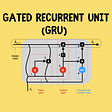# Uncovering the Deep State… of Neural Networks

Deep Learning Math Walk-Through and Code Logic

In the previous blog post we walked through an example where we used a neural network with two neurons and a single hidden layer to produce a (wildly inaccurate) prediction function. As we discussed before, this is fine if two kinks in your function are enough, but if you need more complexity in the functional shape, you have to either add more nodes to your hidden layer or add another hidden layer (or both!) In this post, we will take a look at what happens when we decide to introduce a second hidden layer with the same number of nodes, and walk through the math as well to make it a little more real.

In my theoretical overview of deep learning, we presented an overview of a deep learning neural network that looked like this:

We already know what happens up to the first activation layer, but here is where the deep learning path diverges. Rather than immediately summing up the activation node outputs, these outputs get individually passed through another set of weight and bias calculations — one for each node in the next layer (so in our example, two sets) and these results are combined and fed as inputs into the next activation layer.

Back to our working example: We start with an output from the first set of weights/biases (calculated using inner products of random weights, actual data, and random bias values):

This gets passed through the ReLU activation layer:

In our single hidden layer, this output got converted directly into the final predictions. But now, instead of a single set of weights and bias values, we apply two sets of weights and bias values to each neuron, designated in our diagram as a grouping of weights 3 and 5 (w35 in matrix form), and then 4 and 6 (or w46). Let’s generate those:

And generate the random bias values (b3 and b4 in our diagram):

Now we’re set up and ready to do the math!

# Plug ’n’ Chug

We take our output from the first neuron and multiply it by our two weights, compute the sums for each prediction at this stage, and add the appropriate offset values. Let’s demonstrate the top neuron one step at a time:

If we were to diagram this for visualization purposes, this would be a function with two kinks in it, just like the final prediction in the single-layer example.

In the single-layer example, this would be our final predictions, but now we need to do the same computations for the second node in the hidden layer and pass those values along. The single step would look like this:

We have logically created two functions, each with two kinks (just like our single-layer neural network from the previous post), as the outputs / predictions of the first hidden layer. Now these predictions get combined into a single matrix so that we can do a single multiplication step in the next layer:

At this point, we should be in very familiar territory —it’s basically a repeat of the previous steps. We run this output through our activation function:

…create a set of random weights and the final offset:

…and create our final set of outputs, which is now our final prediction for this forward pass, which is based on a function that — if diagrammed — would contain four separate kinks:

# A Programmatic Approach

Let’s create a couple of functions to simplify our lives a bit. First, let’s code our activation node as a function so that we can simply call ReLU() and provide it with a matrix, and it will convert it and return the activated version with no negative values:

Next we’ll define a function that does the math for us to apply our weights to the previous output. Note that I’ve added in some logic to generate random weights on the fly if they’re missing, but in reality the weights should all be calculated for each layer beforehand and tracked outside of this function, since they’re going to be modified as part of the gradient descent process down the road.

As a sanity check, compare this to our long-form result from out3_final:

So far so good!

Now we just need to replicate this step for the same output, but different weights and biases per activation node in the next layer. We could simply run TheMaths again:

…and then use np.vstack() to recombine them for the final processing, or we could just put all of that work into another function as well:

We could now have 10 hidden layers with two nodes each and reuse the same function code for each one, supplying the appropriate weight and bias values along the way.

Now we have a single line of code that passes in the outputs from a previous hidden layer, plus (initially) randomly generated weights and biases. This gets us all the way from our initial out1 to the out5 stage of the previous longform example with a single command. Check the output below and keep me honest!

Now for the final predictions, we need just a single output so we reuse our TheMaths function to generate the final array, and then add the final offset value.

Note here that we’ve encapsulated everything in the deep network from the first output of the input layer to the final prediction.

If we wanted to add more layers, you could simply keep nesting the Relu(TwoNode(…)) pattern as many times as necessary. Of course, there are more sophisticated ways of doing this. You’d probably want to consider some kind of recursive function which simply called itself until the number of hidden layers had been reached. But from a conceptual standpoint, the framework of the math logic is now complete.

Now all that’s left is to modify the approach based on different parameters. We might want to allow the user to more loosely configure layers and nodes, for example, or perhaps modify the activation function to avoid zero values (perhaps using a modification known as the “leaky ReLU”). We also need to determine how to generate and track the random weights and bias values. There are no shortage of suggested approaches, and someday this might be a topic of interest for this blog. Today, however, is not that day. :)

While this all gets a bit involved, at the end of the day it’s actually a straightforward process with a lot of repetitive logic. Understanding how it works means you understand, at a very basic level, how a deep neural network makes predictions. Again, in the first pass you’re all but guaranteed for these to be very, very bad predictions, so we still need to figure out how the whole back propogation / gradient descent thing works. But understanding how deep neural networks actually compute their values is good enough for now. We’ll get back to back propogation.

--

--

--

## More from MLearning.ai

Data Scientists must think like an artist when finding a solution when creating a piece of code. ⚪️ Artists enjoy working on interesting problems, even if there is no obvious answer ⚪️ linktr.ee/mlearning 🔵 Follow to join our 18K+ Unique DAILY Readers 🟠

## Dimension Reduction using Isomap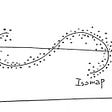## Neural Networks for Steady-State Fluid Flow Prediction: Part 2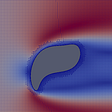## Signal Modeling Using Recurrent Neural Networks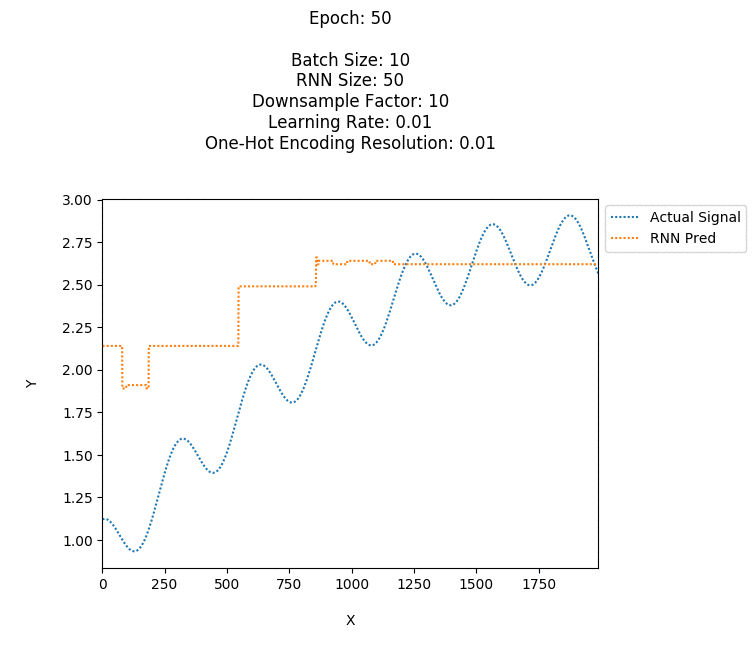## Build Your First Computer Vision Project — Dog Breed Classification## Final Project-Selecting Models to Predict CHD## PyTorch Lightning Bolts — From Boosted Regression on TPUs to pre-trained GANs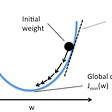## Neural Networks gone wild! They can sample from discrete distributions now!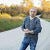## Jason Eden

Data Science & Cloud nerd with a passion for making complex topics easier to understand. All writings and associated errors are my own doing, not work-related.

## Let’s Learn: Neural Nets #1## Neural Networks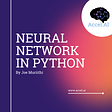## GRU Recurrent Neural Networks — A Smart Way to Predict Sequences in Python